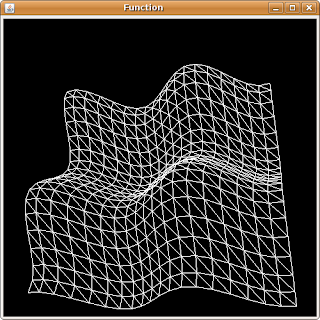## Saturday, March 31, 2007

### Plotting 3D functions with Groovy and Java3D

In this post I'm going to show a little demo that plots 3D functions by using Groovy and Java3D.

For this demo I'm going to use the Java3D builder created for previous posts.

The TriangleArray class will be used to create the grid to plot the function . Two triangles will be create for each set of 4 points. For future posts I'm going to try to change this to support TriangleStripArray or QuadArray.

In order to create each coordinate of the plane, a function that maps between the iteration number and the real coordinate to be plotted is required. In order to do this the createMapFunc was created, this function returns a function that maps between two coordinates.

`Closure createMapFunc(int x1,int x2,double y1,double y2) { double m = (y2 - y1)/(x2 - x1); double b = y1 - m*x1; return { x -> m*x + b}}Closure createFloatMapFunc(int x1,int x2,double y1,double y2) { Closure f = createMapFunc(x1,x2,y1,y2); return { (float)f(it)};}`

Given this we can now create the TriangleArray data required to plot the function:

`Geometry createGeometry(Closure f,double min,double max,int gridSize) { Closure fInter = createFloatMapFunc(0,gridSize-1,min,max); TriangleArray ta = new TriangleArray(((gridSize-1)**2)*6, TriangleArray.COORDINATES |TriangleArray.NORMALS ); int idx = 0; float x,y,z; for ( iY in (0..(gridSize-2))) { for( iX in (0..(gridSize-2))) { // First triangle x = fInter(iX); y = fInter(iY); z = (float) f(x,y); ta.setCoordinate(idx,new Point3f(x,y,z)); x = fInter(iX+1); y = fInter(iY); z = (float) f(x,y); ta.setCoordinate(idx+1,new Point3f(x,y,z)); x = fInter(iX); y = fInter(iY+1); z = (float) f(x,y); ta.setCoordinate(idx+2,new Point3f(x,y,z)); idx += 3; // Second triangle x = fInter(iX+1); y = fInter(iY); z = (float) f(x,y); ta.setCoordinate(idx,new Point3f(x,y,z)); x = fInter(iX+1); y = fInter(iY+1); z = (float) f(x,y); ta.setCoordinate(idx+1,new Point3f(x,y,z)); x = fInter(iX); y = fInter(iY+1); z = (float) f(x,y); ta.setCoordinate(idx+2,new Point3f(x,y,z)); idx += 3; } } return ta;}`

The function to be plotted is a parameter to the createGeometry function.

For this example the following function will be used:

``def f = { x,y -> Math.cos(x+y)*Math.sin(x-y)}``

Now that we have the code to generate the 3D function geometry we can create the Java3D/Swing required elements by using the Swing, Java3D and SimpleUniverse builders.

``SwingBuilder sb = new SwingBuilder();SimpleUniverseBuilder sub = new SimpleUniverseBuilder();Java3dBuilder jb = new Java3dBuilder();         JFrame aFrame = sb.frame(title:"Function",size:[500,500]){    panel(layout: new FlowLayout()) {        thePanel = panel(preferredSize:[500,500],                         layout:new BorderLayout())            } }SimpleUniverse univ =     sub.simpleUniverse() {         viewingPlatform(nominalViewingTransform:true) {            orbitBehavior(                          flags:OrbitBehavior.REVERSE_ALL,                          bounds:new BoundingSphere(                                            new Point3d(0.0,0.0,0.0),                                             100.0))         }         viewer() {           view(minFrameCycleTime:5)                          }    }thePanel.add(univ.getCanvas(),BorderLayout.CENTER);BranchGroup bg =     jb.branchGroup() {         transformGroup(               capability:                       TransformGroup.ALLOW_TRANSFORM_WRITE) {        shape3d(geometry:createGeometry(f,-2.5,2.5,20),                 appearance:polyAppearance())    }}bg.compile();univ.addBranchGraph(bg);aFrame.show()``

The result of running the program looks like this:As a last detail, the appearance of the surface is created with the createPolyAppearance function:

``Appearance polyAppearance() {    Appearance app = new Appearance();    PolygonAttributes pa = new PolygonAttributes();    pa.setPolygonMode(PolygonAttributes.POLYGON_LINE);    app.setPolygonAttributes(pa);    return app;}``

Code for this experiment can be found here.

In future posts I'm going to try to add more features, like materials applied to the surface geometry, axis lines, etc.